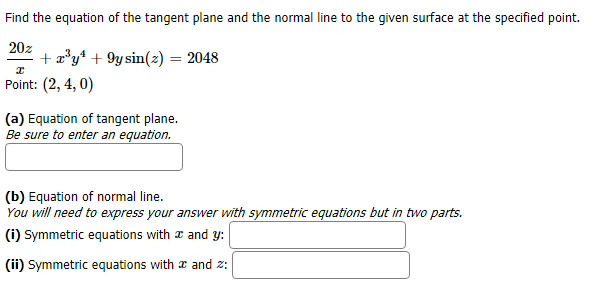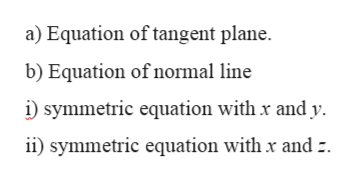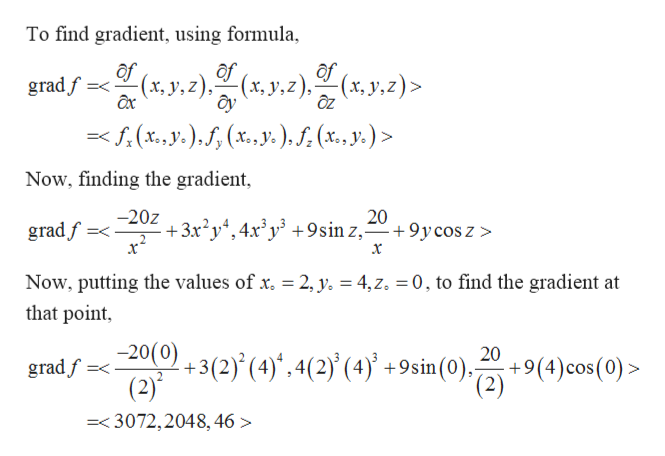# Find the equation of the tangent plane and the normal line to the given surface at the specified point.20zay9ysinz) = 2048Point: (2, 4, 0)(a) Equation of tangent plane.Be sure to enter an equation.(b) Equation of normal lineYou will need to express your answer with symmetric equations but in two parts.(i) Symmetric equations withand y(ii) Symmetric equations with æ and z

Question
25 viewshelp_outlineImage TranscriptioncloseFind the equation of the tangent plane and the normal line to the given surface at the specified point. 20z ay9ysinz) = 2048 Point: (2, 4, 0) (a) Equation of tangent plane. Be sure to enter an equation. (b) Equation of normal line You will need to express your answer with symmetric equations but in two parts. (i) Symmetric equations with and y (ii) Symmetric equations with æ and z fullscreen
check_circle

Step 1

Given: -

Step 2

To find: -help_outlineImage Transcriptionclosea) Equation of tangent plane b) Equation of normal line symmetric equation with x and y i symmetric equation with x and : fullscreen
Step 3

Calculation: -

Pa...help_outlineImage TranscriptioncloseTo find gradient, using formula of (x, y, z), (x, y,z)> (x,y,z), gradf = -20z +3x2y4,4x'y +9sin z, gradf 2, y. = 4, z. 0, to find the gradient at Now, putting the values of x. that point 20(0) +3(2) (4)',4(2) (4)* +9sin(0)-2) (2) 20 +9(4)cos(0)> gradf =3072,2048,46> fullscreen

### Want to see the full answer?

See Solution

#### Want to see this answer and more?

Solutions are written by subject experts who are available 24/7. Questions are typically answered within 1 hour.*

See Solution
*Response times may vary by subject and question.
Tagged in

### Derivative